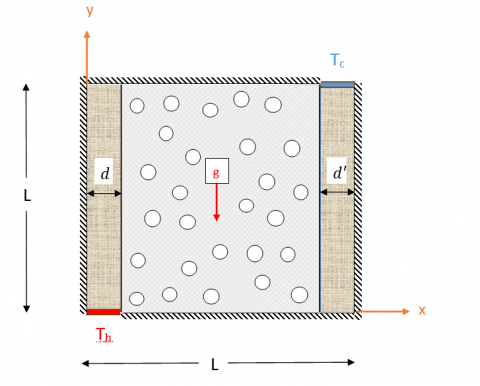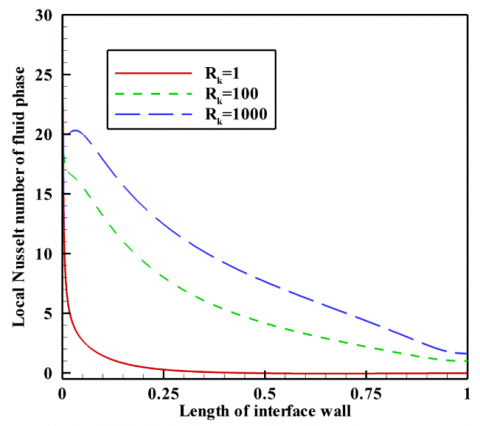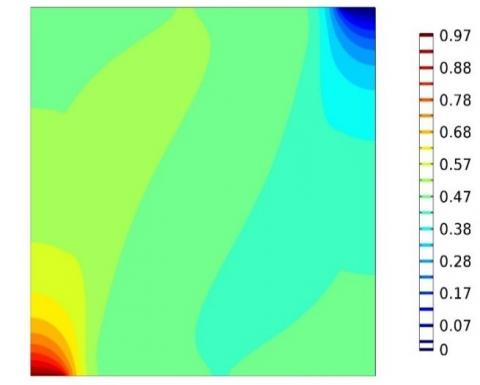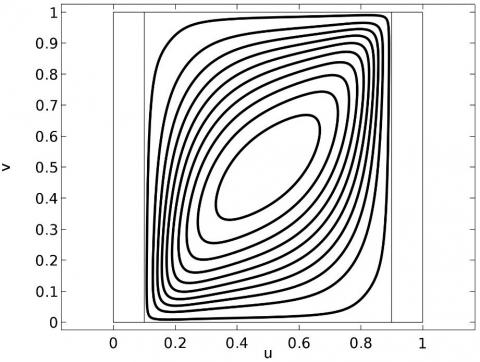# Non-equilibrium Natural Convection Flow through a Porous Medium

Non-equilibrium Natural Convection Flow through a Porous Medium
Civil Engineering Department, College of Engineering and petroleum, Kuwait University, P.O. Box 5969, Safat, Kuwait

Corresponding Author Email:
jalhumoud@yahoo.com
Page:
163-169
|
DOI:
https://doi.org/10.18280/mmep.060202
3 March 2019
|
Accepted:
25 May 2019
|
Published:
20 June 2019
| Citation

OPEN ACCESS

Abstract:

The problem of conjugate natural convection flow in a layer of porous media confined by two vertical thermally-conductive thick surfaces is studied. The local thermal non-equilibrium approach is employed for modelling the porous layer allowing for a difference in temperature between the porous structure and the fluid inside it. The basic equations for this investigation are transformed into a set of dimensionless equations. Then, the resulting equations are solved numerically by employing the finite element method. The obtained results are compared with published results in the open literature and are found to be in good agreement. The effects of the wall thermal conductivity parameter, fluid and porous interfacial convective heat transfer parameter and the Rayleigh number on the thermal behavior of the cavity are studied and analysed. The results show that increasing all of the above mentioned parameters results in increasing the heat transfer in the cavity.

Keywords:

natural convection, non-equilibrium model, porous layer, porous medium

1. Introduction

Natural convection flow and heat transfer in porous media-filled enclosures have numerous applications including geothermal heat recovery, heat sinks, the phase change energy-storage devices, filter media, underground energy storage, and nuclear waste disposal sites. Owing the fact that natural convection in porous media finds important industrial and engineering applications, various aspects of heat transfer in porous media have been explored by many researchers in recent years.

Many of the available research works on this topic have assumed Local Thermal Equilibrium (LTE) between the porous structure and the fluid inside it. In this case, only one heat equation would represent the thermal behavior of the porous medium and the fluid filling it. Employing the thermal equilibrium model of porous media, Basak et al. [1-4] addressed different aspects of boundary conditions on the natural convection of a fluid-saturated porous medium in a cavity. Grosan et al. [5, 6] explored the effect of a magnetic field on the free convection heat transfer in an enclosure. Sun and Pop  studied the natural convection in a triangular cavity. Very recently, Mehryan et al.  considered the problem of free convection heat transfer of hybrid nanofluids in an enclosure filled with a porous medium using the LTE model of porous media.

The porous media Local Thermal Non-Equilibrium (LTNE) model is a two heat equations model. In this model, the thermal behavior of the porous structure and the fluid filling it are introduced by two temperature variables. Hence, the temperature of the porous structure and the fluid inside it can be different. Additionally, the thermal interaction between the porous medium and the saturating fluid filling its pores is taken into account using some interfacial convective heat transfer coefficients. Taking into account the LTNE model, Ghalambaz et al.  analyzed the free convective heat transfer in a parallelogrammic enclosure. Zargartalebi et al.  investigated the natural convective flow and heat transfer in a cavity. In , the cavity was partially occupied by a porous fin. The porous fin was modeled as a LTNE porous medium.

Considering the problem of conjugate heat transfer along with the porous media LTNE model, the transfer of heat at the solid surface-porous matrix interface is a complex phenomenon. In the conjugate heat transfer, the porous medium is connected to a thermally conductive solid wall. From the physical point of view, there are several pathways for the transfer of heat between the surface or wall and the porous matrix structure and the fluid inside the pores. The heat from the wall will be divided at least into two pathways. One such pathway is the transfer of heat between the wall and the porous structure due to heat conduction. The other is the heat transfer due to convection between the fluid filling the porous media and the wall surface. There are also thermal interactions between the fluid inside the pores and the porous matrix which affect the heat transfer pathways. Employing the conjugate heat transfer and the porous media LTNE model, Saeid  modeled the conjugate heat transfer in a layer of porous media placed in a cavity. Zargartalebi et al.  considered the unsteady fee convection of a nanofluid in an enclosure filled with porous media. Tahmasebi et al.  investigated the natural convection of nanofluids in an enclosure filled with a solid wall layer, a layer of porous media, and a layer of clear flow.

Following the study of Zargartalebi et al.  and Tahmasebi et al. , the present study aims to address the conjugate free convective heat transfer behavior in an enclosure with two solid thick surfaces of walls in which the heat enters the enclosure from the bottom of the thick surface and leaves the enclosure from the top of another surface.

2. Mathematical model

2.1 Model description

Consider a square cavity with length L containing a layer of metallic foam porous media and sandwiched between two thick thermally-conductive surfaces. The thicknesses of the walls are d and d'. The schematic diagram of the problem and the coordinate system are illustrated in Figure 1.

It is assumed that the temperature differences inside the porous domain are limited, and hence, all of the thermophysical properties are assumed to be constant except the variation in density due to temperature change for which the Boussinesq approximation is applicable. The flow in the porous domain is modeled as a Darcy-Brinkman flow.

## 111-.pngFigure 1. Schematic diagram of the problem and the coordinate system

2.2 Governing equations

By considering the modeling assumptions, the governing equations for mass, linear momentum, energy of the fluid inside the porous domain, energy inside the porous structure and the energy in the thick solid surfaces can be written as follows [12, 13]:

$\frac{\partial u}{\partial x}+\frac{\partial v}{\partial y}=0$  (1)

$\frac{\rho_{f}}{\varepsilon^{2}}\left(u \frac{\partial u}{\partial x}+v \frac{\partial u}{\partial y}\right)=-\frac{\partial P}{\partial x}+\frac{\mu_{f}}{\varepsilon}\left(\frac{\partial^{2} u}{\partial x^{2}}+\frac{\partial^{2} u}{\partial y^{2}}\right)-\frac{\mu_{f}}{\kappa} u$  (2)

$\frac{\rho_{f}}{\varepsilon^{2}}\left(u \frac{\partial v}{\partial x}+v \frac{\partial v}{\partial y}\right)=-\frac{\partial P}{\partial y}+\frac{\mu_{f}}{\varepsilon}\left(\frac{\partial^{2} v}{\partial x^{2}}+\frac{\partial^{2} v}{\partial y^{2}}\right)-\frac{\mu_{f}}{\kappa} v+\rho_{j} \beta\left(T_{f}-T_{c}\right) g$  (3)

$\frac{1}{\varepsilon}\left(u \frac{\partial T_{f}}{\partial x}+v \frac{\partial T_{f}}{\partial y}\right)=\alpha_{f}\left(\frac{\partial^{2} T_{f}}{\partial x^{2}}+\frac{\partial^{2} T_{f}}{\partial y^{2}}\right)+\frac{h_{t}}{\varepsilon(\rho c)_{f}}\left(T_{s}-T_{f}\right)$  (4)

$0=\alpha_{s}\left(\frac{\partial^{2} T_{s}}{\partial x^{2}}+\frac{\partial^{2} T_{s}}{\partial y^{2}}\right)+\frac{h_{f s}}{(1-\varepsilon)(\rho c)_{s}}\left(T_{f}-T_{s}\right)$  (5)

$0=\left(\frac{\partial^{2} T_{w}}{\partial x^{2}}+\frac{\partial^{2} T_{w}}{\partial y^{2}}\right)$  (6)

where u and v denote the fluid velocities in the x and y directions, respectively. Here, P and T are the pressure and temperature, respectively. The subscripts of f, s and w indicate the fluid, the porous media and the surface or wall, respectively.

The symbols of µ, ρ, β, α, cp, κ, and ε denote the dynamic viscosity, the density, volumetric thermal expansion coefficient, thermal diffusivity, specific heat capacity, permeability of the porous medium and   porosity of the porous medium, respectively. Finally, hfs indicates the coefficient of the convective heat transfer between the porous structure and the fluid inside it.

Based on the problem description, the boundary conditions at the walls and the wall-porous interface are represented as:

at the hot wall: $T=T_{h}$  (7)

at the cold wall: $T=T_{c}$  (8)

at the insulated walls: $\quad \partial T / \partial n=0$  (9)

at the walls - porous interface: $k_{w} \partial T_{w} / \partial n=\varepsilon k_{f} \partial T_{f} / \partial n+(1-\varepsilon) k_{s} \partial T_{s} / \partial n$ and $T_{f}=T_{s}=T_{w}$  (10)

at the walls: $u=v=0$  (11)

where k is the thermal conductivity.

By applying the following dimensionless parameters:

$X=\frac{x}{L}, Y=\frac{y}{L}, D=\frac{d}{L}, D^{\prime}=\frac{d^{\prime}}{L}, U=\frac{u L}{\alpha_{f}}, V=\frac{v L}{\alpha_{f}}, P=\frac{P L^{2}}{\rho_{f} \alpha_{f}^{2}}$  $\theta_{f}=\frac{T_{f}-T_{c}}{T_{h}-T_{c}}, \theta_{s}=\frac{T_{s}-T_{c}}{T_{h}-T_{c}}, \theta_{\mathrm{w}}=\frac{T_{w}-T_{c}}{T_{h}-T_{c}}$  (12)

the governing equations (1)-(6) along with the boundary conditions (7)-(11) are transformed into the following non-dimensional form:

$\frac{\partial U}{\partial X}+\frac{\partial V}{\partial Y}=0$  (13)

$\frac{1}{\varepsilon^{2}}\left(U \frac{\partial U}{\partial X}+V \frac{\partial U}{\partial Y}\right)=-\frac{\partial P}{\partial X}+\frac{\operatorname{Pr}}{\varepsilon}\left(\frac{\partial^{2} U}{\partial X^{2}}+\frac{\partial^{2} U}{\partial Y^{2}}\right)-\frac{\operatorname{Pr}}{D a} U$  (14)

$\frac{1}{\varepsilon^{2}}\left(U \frac{\partial V}{\partial X}+V \frac{\partial V}{\partial Y}\right)=-\frac{\partial P}{\partial Y}+\frac{\operatorname{Pr}}{\varepsilon}\left(\frac{\partial^{2} V}{\partial X^{2}}+\frac{\partial^{2} V}{\partial Y^{2}}\right)-\frac{\operatorname{Pr}}{D a} V+R a \operatorname{Pr} \theta_{f}$  (15)

$\frac{1}{\varepsilon}\left(U \frac{\partial \theta_{f}}{\partial X}+V \frac{\partial \theta_{f}}{\partial Y}\right)=\left(\frac{\partial^{2} \theta_{f}}{\partial X^{2}}+\frac{\partial^{2} \theta_{f}}{\partial Y^{2}}\right)+H\left(\theta_{s}-\theta_{f}\right)$  (16)

$0=\left(\frac{\partial^{2} \theta_{s}}{\partial X^{2}}+\frac{\partial^{2} \theta_{s}}{\partial Y^{2}}\right)+H \times K r\left(\theta_{f}-\theta_{s}\right)$  (17)

$0=\left(\frac{\partial^{2} \theta_{w}}{\partial X^{2}}+\frac{\partial^{2} \theta_{w}}{\partial Y^{2}}\right)$  (18)

where Pr is the Prandtl number, Da is the Darcy number, Ra is Rayleigh, H is the convection interaction, and kr is the thermal conductivity ratio of the fluid and the porous medium. These parameters are defined as:

$\operatorname{Pr}=\frac{\mu_{f}}{\rho_{f} \alpha_{f}}, D a=\frac{\kappa}{L^{2}}, R a=\frac{g \rho \beta\left(T_{h}-T_{c}\right) L^{3}}{\mu_{f} \alpha_{f}}, H=\frac{h_{f 5} L^{2}}{\varepsilon k_{f}}, K r=\frac{\varepsilon k_{f}}{(1-\varepsilon) k_{s}}$  (19)

The non-dimensional boundary conditions can be written as:

at the hot wall : $\theta=1$  (20)

at the cold wall: $\theta=0$  (21)

at the insulated walls: $\partial \theta / \partial n=0$  (22)

at the walls - porous interface: $\partial \theta_{f} / \partial n=R_{k} \partial \theta_{w} / \partial n-K_{r}^{-1} \partial \theta_{s} / \partial n$  (23)

at the walls: $\quad U=V=0$  (24)

where Rk=kw/(εkf) is the thermal conductivity ratio of the solid wall and the fluid.

The key characteristics of the present study are the Nusselt number in the fluid and the porous structure at the interface of the wall and the porous medium which are introduced as:

$N u_{f}=\left(\partial \theta_{f} / \partial n\right)_{X=D}$     $N u_{s}=-\left(\partial \theta_{s} / \partial n\right)_{X=D}$  (25)

The average Nusselt number can be evaluated by integrating the local Nusselt number along with the interface as follows:

$N u_{f}=-\int_{0}^{1}\left(\partial \theta_{f} / \partial n\right)_{X=D} dy$     $N u_{s}=-\int_{0}^{1}\left(\partial \theta_{s} / \partial n\right)_{X=D} dy$  (26)

3. Numerical Method, Grid Check and Validation

The finite element method was employed to integrate the governing equation (13)-(18) along with the boundary conditions (20)-(24). The governing partial differential equations were first put in the weak form and then solved iteratively as a fully coupled set of equations. A structured non-uniform grid was utilized to discrete the governing equations. The grid was stretched near the walls with a ratio of 10. Hence, the density of the grid points was higher near the surfaces in order to capture the high gradients in these regions. The residual error for the iterations was set to 10-8. More details about the implementation method can be found in .

In order to assess the accuracy of the utilized grid, the numerical calculations were repeated for different grid sizes, and the outcomes are monitored and summarized in Table 1. As seen, the grid size of 160×160 provides sufficient accuracy for a graphical demonstration of the results and most of the engineering applications. Thus, the outcome of the present study are reported for this grid size.

Table 1. The grid-check results for Average Nusselt number

 The grid sizea 100×100 160×160 200×200 Nuf 0.517 0.522 0.525 Nus 0.175 0.174 0.174

a. $D a=10^{-3}, \operatorname{Pr}=7.2, D=D^{\prime}=0.1, H=10, \varepsilon=0.5, R a=10^{6}$

In order to check the correctness and accuracy of the present numerical code, the results were compared with some related works available in the literature [1, 8] and were found in good agreement. Moreover, a comparison between the results of the present study and the results of Saeid  is provided in Table II. Saeid  addressed the convection heat transfer in a cavity filled with porous media and heated from side walls for a Darcy flow. Considering a very high value of Darcy number, the governing equations of the present study were reduced to the case of Darcy flow. In this case, the Rayleigh number would be the Darcy-Rayleigh number (Da×Ra), and the solution would be independent of the Prandtl number. Table 2 shows good agreement between the results of Saeid .

Table 2. Comparison between the results of the present study and the literature results

 Rk Type Average Nusselt numbera Present study Saeid  0.01 Nuf 0.343 0.326 Nus 0.112 0.110 1 Nuf 2.904 2.814 Nus 0.429 0.418 10 Nuf 9.840 9.887 Nus 1.013 1.010

a. $D=D^{\prime}=0.1, H=1, K^{*}=1, D a \times R a=10^{3}$
4. Results and Discussion

In the present study, the default values of the non-dimensional parameters were adopted as D=D'=0.1, H=1, Kr=1, Da=10-3, Ra=105, Pr=7.2, Rk=1. Otherwise the value of the non-dimensional parameter is stated.

Figure 2 shows the local Nusselt number of the fluid phase along the hot interface for various values of the wall thermal conductivity ratio Rk. Figure 3 depicts the heat distribution in the wall and solid porous matrix in the form of non-dimensional temperature contours for various values of Rk.

As seen, next to the bottom of the wall, the local Nusselt number is high, and then, it decreases along the wall toward the upward direction. For the low wall thermal conductivity ratio of Rk=1, the Nusselt number at the bottom of the wall is high, and then it drops rapidly. This is due to the low thermal conductivity of the solid wall. The heat at the hot boundary located at the bottom of the thick wall enters the wall and moves in an upward direction as a result of the thermal conduction mechanism. A portion of the heat goes into the fluid due to the convection at the interface of the wall and the porous domain. As the thermal conductivity of the wall is low, the heat can only be distributed in the bottom portion of the wall, and this is where the heat transfer between the wall and the fresh cold fluid inside the pores occurs. The corresponding isotherms in the wall are depicted in Figure 3 (a). As seen, only the bottom part of the hot wall is hot and the other parts are at low temperatures near to that of the porous matrix next to the wall.

When the thermal conductivity of the wall increases, i.e., a higher wall thermal conductivity ratio of Rk=100, a better distribution of heat in the wall occurs, and a more extended portion of the wall is hot. The temperature distribution in the wall for this case is depicted in Figure 3 (b). As seen, up to the middle of the wall is at a high temperature. Thus, in most places of the wall, the local Nusselt number is high, the outcome of which is in agreement with the results of Figure 2. Finally, when the thermal conductivity ratio of the wall increases to Rk=1000, almost all of the solid wall is at a high temperature, and consequently, the local Nusselt number along the wall is high. At the top of the wall, both the fluid, porous matrix and the wall itself are at a high temperature, and hence, a gradual drop of the local Nusselt number can be expected in this region. This outcome is also in agreement with the results of Figure 2.

Figure 4 shows the streamlines corresponding to the cases of Figures 2 and 3. As seen, the increases of Rk from 1 to 100 do not induce a notable change in the streamlines. However, with the increase of Rk from 100 to 1000, the change in the patterns of streamlines is more notable. In the case of low values of Rk, the hot part of the wall is at the bottom, and the streamlines are a bit denser at the left bottom of the cavity as depicted in Figs. 4 (a) and (b). However, with the increase of the wall thermal conductivity, all of the wall is at a high temperature, and the streamlines are in an almost normal form.

## 2.pngFigure 2. Average Nusselt number of the fluid phase

## 3a-.jpg(a): Rk=1

## 3b-.jpg(b): Rk=10

## 3c-.jpg(c): Rk=100

Figure 3. The wall and porous matrix isotherms for various values of the wall thermal conductivity ratio (Rk)

## 4a-.jpg(a): Rk=1

## 4b-.jpg(b): Rk=10

## 4c-.jpg(c): Rk=100

Figure 4. The streamlines for various values of the wall thermal conductivity ratio (Rk)

Figure 5 shows the average Nusselt number of the fluid phase as a function of porous-fluid interface convection H and the Rayleigh number. As seen, the increase of the Rayleigh number from 104 to 105 does not affect the average Nusselt number. This is because the Darcy number is as small as Da=10-3, and hence, the Darcy-Rayleigh number is in the range of 10-100. In this case, the heat transfer is a conduction-dominant form, and the variation of the Rayleigh number does not change the fluid flow. For the larger Rayleigh number of Ra=106 (Darcy-Rayleigh number=1000), the convection-mechanisms strengthen, and the changes in the average Nusselt number are notable. Figure 6 illustrates the effect of interfacial convection interaction between the porous structure and the fluid inside the pores, H. The increase of this parameter is related to the increase of thermal interaction between the porous structure and the fluid inside the pores.

## 5.pngFigure 5. The average Nusselt number as a function of porous-fluid interface convection and Rayleigh number

## 6a.png(a): H=0.01

## 6b.png(b): H=10

## 6c.png(c): H=50

Figure 6. The wall and porous matrix isotherms (a): H=0.01, H=10 and H=50

For very high values of H, the temperature of the porous structure and the fluid inside pores nearly equalize, and the LTNE model can be reduced to the LTE model. As seen with the increase of H, the temperature profiles inside the porous space change slightly. Figure 5 illustrates the smooth increase of the fluid Nusselt number with the increase of H. Indeed, with the increase of H, the porous matrix would help the fluid to increase the heat transfer rate in the cavity.

5. Conclusion

The problem of conjugate flow and heat transfer in an enclosure containing a porous medium was addressed using the LTNE model. The cavity or enclosure’s walls were thick and thermally conductive. The heat was assumed to enter the cavity through the bottom of a wall and to leave the cavity through the top of the other wall. A two-temperature LTNE model of porous media was employed to represent the temperature of the fluid phase and the porous structure. The conjugate heat transfer at the interface of the solid surfaces and the porous domain were computed using the continuity of temperature and heat balance. The effects of wall thermal conductivity (wall thermal conductivity ratio), the Rayleigh number and the porous-fluid interface convection parameter (H) on the free convection heat transfer in the enclosure were addressed. The primary outcomes of the present study can be summarized as follows:

• The increase of the wall thermal conductivity ratio (Rk) increases the local Nusselt number in the fluid phase.
• The increase of the porous-fluid interface convection parameter (H) slightly increases the average Nusselt number of the fluid phase.
• The increase of the Rayleigh number increases heat transfer. However, the increase of the Rayleigh number for small values of the Darcy-Rayleigh number (lower than 100) is almost ineffective.

In the present investigation, the effect of the thickness of the walls and the thermal conductivity of the porous medium on the natural convective heat transfer were not studied, thus providing a subject for future studies.

Acknowledgment

The author is very thankful and grateful to the posthumous Professor Ismail Esen (peace be upon him) for his guidance and suggestions. In addition, the author is very thankful to the distinguished reviewers of the journal for their insightful comments and suggestions.

References

 Basak T, Roy S, Paul T, Pop I. (2006). Natural convection in a square cavity filled with a porous medium: Effects of various thermal boundary conditions. International Journal of Heat and Mass Transfer 49(7-8): 1430–1441. http://dx.doi.org/10.1016/j.ijheatmasstransfer.2005.09.018

 Basak T, Roy S, Takhar H. (2007). Effects of nonuniformly heated wall(S) on a natural-convection flow in a square cavity filled with a porous medium. Numerical Heat and Transfer Part A Appl. 51(10): 959-978. http://dx.doi.org/10.1080/10407790601128600

 Basak T, Roy S, Singh A, Pop I. (2009). Finite element simulation of natural convection flow in a trapezoidal enclosure filled with porous medium due to uniform and non-uniform heating. International Journal of Heat and Mass Transfer 52(1–2): 70-78. http://dx.doi.org/10.1016/j.ijheatmasstransfer.2008.03.032

 Basak T, Roy S, Paul T, Pop I. (2006). Natural convection in a square cavity filled with a porous medium: Effects of various thermal boundary conditions. International Journal of Heat and Mass Transfer 49(7-8): 1430–1441. http://dx.doi.org/10.1016/j.ijheatmasstransfer.2005.09.018

 Grosan T, Revnic C, Pop I, Ingham D. (2009). Magnetic field and internal heat generation effects on the free convection in a rectangular cavity filled with a porous medium. International Journal of Heat and Mass Transfer 52(5-6): 1525-1533. http://dx.doi.org/10.1016/j.ijheatmasstransfer.2008.08.011

 Revnic C, Grosan T, Pop I, Ingham D. (2011). Magnetic field effect on the unsteady free convection flow in a square cavity filled with a porous medium with a constant heat generation. International Journal of Heat and Mass Transfer 54(9–10): 1734–1742. http://dx.doi.org/10.1016/j.ijheatmasstransfer.2011.01.020

 Sun Q, Pop I. (2011). Free convection in a triangle cavity filled with a porous medium saturated with nanofluids with flush mounted heater on the wall. International Journal of Thermal Science 50(11): 2141-2153. http://dx.doi.org/10.1016/j.ijthermalsci.2011.06.005

 Mehryan S, Kashkooli F, Ghalambaz M. (2017). Free convection of hybrid Al2O3-Cu water nanofluid in a differentially heated porous cavity. Advances in Powder Technology 28(9): 2295-2305.

 Pop I, Ghalambaz M, Sheremet M. (2016). Free convection in a square porous cavity filled with a nanofluid using thermal non equilibrium and Buongiorno models. International Journal of Numerical Methods and Heat Fluid Flow 26(3-4): 671-684. http://dx.doi.org/10.1108/HFF-04-2015-0133

 Zargartalebi H, Ghalambaz M, Noghrehabadi A. (2016). Natural convection of a nanofluid in an enclosure with an inclined local thermal non-equilibrium porous fin considering Buongiorno’s model. Numer Heat Transf Part A Appl 70(4): 472-485.

 Saeid N. (2007). Conjugate natural convection in a porous enclosure sandwiched by finite walls under thermal nonequilibrium conditions. Journal of Porous Media 11(3): 259–275. http://dx.doi.org/10.1615/JPorMedia.v11.i3.40

 Zargartalebi H, Ghalambaz M, Khanafer K, Pop I. (2017). Unsteady conjugate natural convection in a porous cavity boarded by two vertical finite thickness walls. International Communication Heat and Mass Transfer 81: 218-231. http://dx.doi.org/10.1016/j.icheatmasstransfer.2016.12.016

 Tahmasebi A, Mahdavi M, Ghalambaz M. (2018). Local thermal nonequilibrium conjugate natural convection heat transfer of nanofluids in a cavity partially filled with porous media using Buongiorno’s model. Numerical Heat Transfer Part A Appl 73(4): 254–276.

 Zienkiewicz O, Taylor R. (2005). The finite element method for solid and structural mechanics. Elsevier Butterworth-Heinemann, North America, USA.